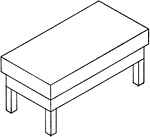### Isometric Of A Carpenter's Bench

Illustration of the isometric of a carpenter's bench.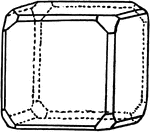### Combination Forms

Illustration showing the combination of various regular systems.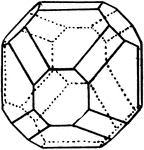### Combination Forms

Illustration showing the combination of various regular systems.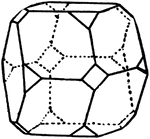### Combination Forms

Illustration showing the combination of various regular systems.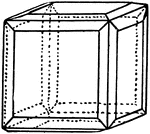### Combination Forms

Illustration showing the combination of various regular systems.### Combination Forms

Illustration showing the combination of various regular systems.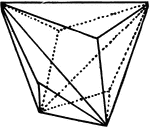### Trigonal Dodecahedron

Illustration showing a trigonal dodecahedron.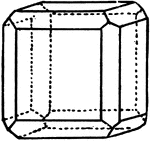### Hexahedron and Dodecahedron

Illustration showing the combination of a hexahedron and an dodecahedron.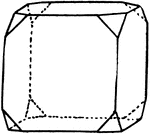### Hexahedron and Octahedron

Illustration showing the combination of a hexahedron and an octahedron.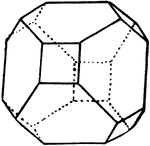### Hexahedron and Octahedron

Illustration showing the combination of a hexahedron and an octahedron.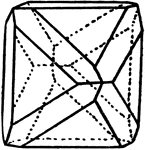### Octahedron and Dodecahedron

Illustration showing the combination of a octahedron and an dodecahedron.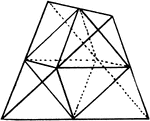### Tetrahedron From Octahedron

Illustration showing the derivation of Tetrahedron from an Octahedron.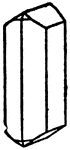### Combination of Prisms

An illustration of a combination formed by different prisms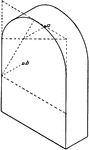### Prism With Semicircular Top

Isometric of a prism with a semicircular top.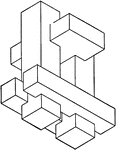### Combination of Geometric Solids

Illustration of model created by combining non regular geometric solids.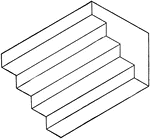### Isometric of Stairs

Illustration of stairs; 3-dimensional view of 4 steps. The staircase can be created by combining/stacking…